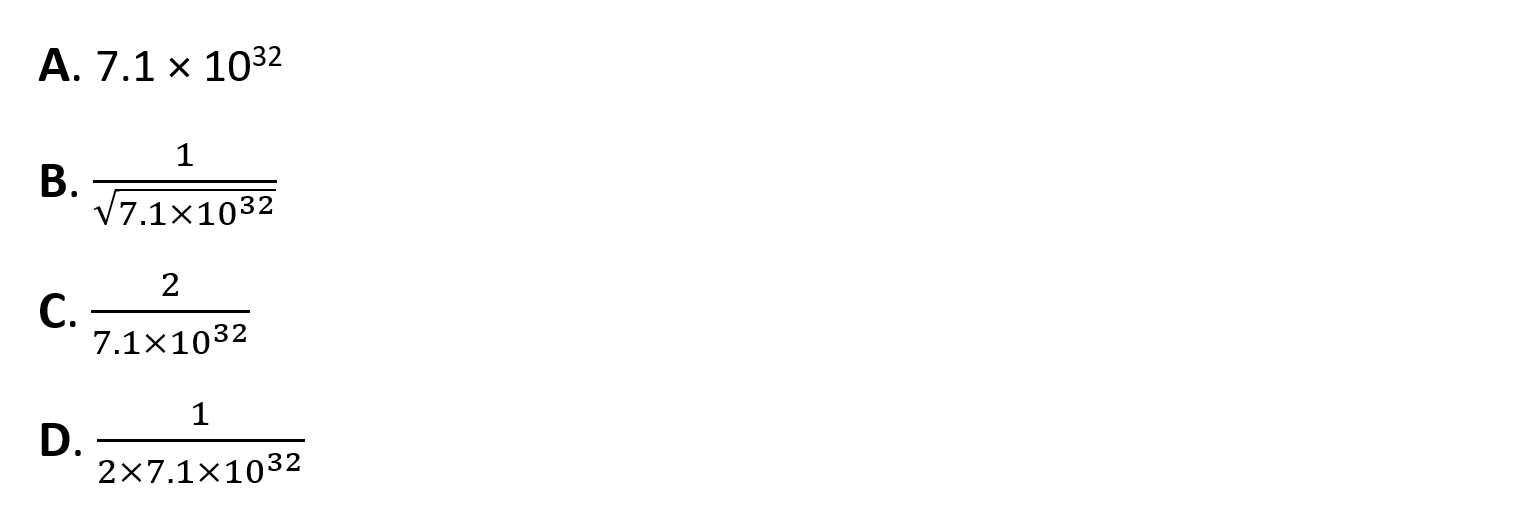# IB DP Chemistry: SL复习笔记7.1.3 Equilibrium Constant Relationships

### Equilibrium Constant Relationships

• In the previous section we saw that the concentrations of the substances are raised to the power of the coefficients from the balanced equation
• This means the Kexpression is dependent on a specific equation
• For example, take the reaction between nitrogen and hydrogen to make ammonia

½N2(g)    +    1½H2(g)  ⇌     NH3(g)

• The Kexpression for this reaction is:• If you double the stoichiometry the equation becomes

N2(g)    +    3H2(g)  ⇌ 2NH3(g)

• The new Kexpression for this reaction is then:• What is the relationship between these two Kc values? You can probably see that when we double the coefficient the new Kc is the square of the original value:• If we reverse the equation:

2NH3(g) ⇌ N2(g)    +    3H2(g)

• Kc  becomes the reciprocal of the original Kvalue:• Test your understanding in the following example:

#### Worked Example

Kc for 2NO2 (g) + F2 (g) ⇌ 2NO2F (g) is 7.1 × 1032What is Kc for the following reaction, at the same temperature?

NO2F (g) ⇌  NO2 (g) + ½F2 (g)The correct option is B.

• The original equation has been reversed and halved, so the Kvalue must be square rooted and inverted to obtain the reciprocal

#### Exam Tip

You must use square brackets in equilibrium constant expressions as they have a specific meaning, representing concentrations. In an exam answer you would lose the mark if you used round brackets